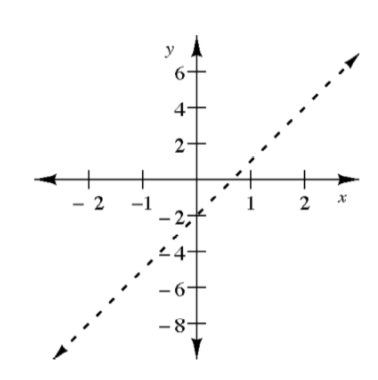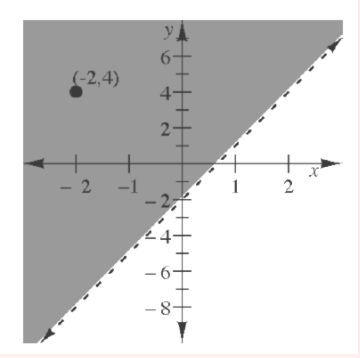### Home > CCG > Chapter Ch7 > Lesson 7.2.2 > Problem7-71

7-71.

On graph paper, graph and shade the solutions for the inequality below.

$y<-\frac{2}{3}x+5$

Example 1

Graph the solutions to the inequality $y>3x-2$.

First, graph the line $y=3x-2$, but draw it dashed since $>$ means the boundary line is not part of the solution. For example, the point $\left(0,-2\right)$ is on the boundary line, but it is not a solution to the inequality because $-2\cancel>3\left(0\right)-2$ or $-2\cancel>-2$.

Next, test a point that is not on the boundary line.
For this example, use the point $\left(-2,4\right)$.

$4>3\left(-2\right)-2, \text{ so } 4>-8$ which is a true statement.

Since the inequality is true for this test point, shade the region containing the point $\left(-2,4\right)$. All of the coordinate pairs that are solutions lie in the shaded region.Use the eTool below to graph and shade the solutions for the inequality.
Click the link at right for the full version of the eTool: CCG 7-71 HW eTool (Desmos)